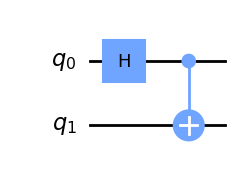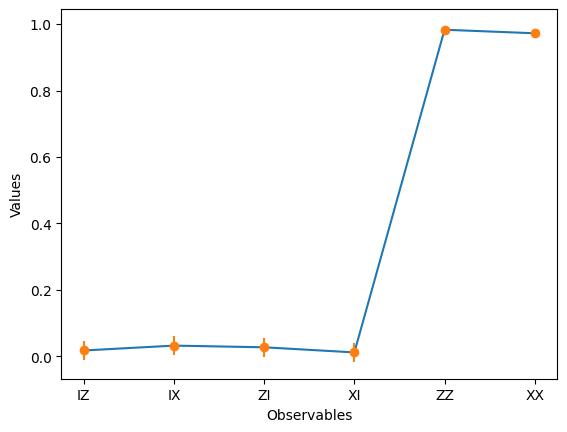# Hello world

This Hello world example creates a simple quantum program and runs it on a quantum system. Begin with following the Install and set up instructions if you haven't already, including the steps to Set up to use IBM Quantum Platform.

We recommend that you use the Jupyter (opens in a new tab) development environment to interact with quantum computers. Be sure to install the recommended extra visualization support (pip install qiskit[visualization]), and note that zsh users need to put 'qiskit[visualization]' in single quotes.

To learn about quantum computing in general, check out the Basics of quantum information course (opens in a new tab) in IBM Quantum Learning.

The four steps to writing a quantum program are

1. Map the problem to a quantum-native format
2. Optimize the circuits and operators
3. Execute using a quantum primitive function
4. Analyze the results

## Step 1. Map the problem to a quantum-native format

In a quantum program, quantum circuits are the native format in which to represent quantum instructions, and operators represent the observables to be measured. When creating a circuit, you'll usually create a new QuantumCircuit object, then add instructions to it in sequence.

The following code cell creates a circuit that produces a Bell state, which is a specific two-qubit entangled state.

Note: bit ordering

Qiskit uses the LSb 0 bit numbering where the $n^{th}$ digit has value $1 \ll n$ or $2^n$. Because we usually write numbers on paper with the most significant digits to the left and the least significant digits to the right (in the Hindu-Arabic system used in most of the world), this has the consequence that the bits are labeled with indices increasing from right to left. This LSb 0 convention makes mathematics easier and is the most commonly used for modern digital electronics, although the opposite convention MSb 0 is also found in some domains. Converting the $i^{th}$ index between LSb 0 and MSb 0 conventions on an $n$-bit register is as simple as $i \rightarrow n-i-1$. This differs across authors and software packages, so be aware!

 :
from qiskit import QuantumCircuit

# Create a new circuit with two qubits (first argument) and two classical
# bits (second argument)
qc = QuantumCircuit(2)

qc.h(0)

# Perform a controlled-X gate on qubit 1, controlled by qubit 0
qc.cx(0, 1)

# Return a drawing of the circuit using MatPlotLib ("mpl"). This is the
# last line of the cell, so the drawing appears in the cell output.
# Remove the "mpl" argument to get a text drawing.
qc.draw("mpl")

Output:See QuantumCircuit in the documentation for all available operations.

The following code cell uses the quantum_info package to create the two-qubit Pauli operator Z on qubit 1 and Z on qubit 2. If the state is entangled, then the correlation between qubit 1 and qubit 2 is one.

 :
from qiskit.quantum_info import Pauli

ZZ = Pauli('ZZ')
ZI = Pauli('ZI')
IZ = Pauli('IZ')
XX = Pauli('XX')
XI = Pauli('XI')
IX = Pauli('IX')

## Step 2. Optimize the circuits and operators

For this example, the circuit the operators are simple, so no optimizations are needed.

## Step 3. Execute using a quantum primitive function

Quantum computers can produce random results, so you'll often want to collect a sample of the outputs by running the circuit many times. You can estimate the value of the observable using the Estimator class. Estimator is one of our two primitives; the other is Sampler, which can be used to get data from a quantum computer.

 :
from qiskit_ibm_runtime import QiskitRuntimeService, Estimator, Options

service = QiskitRuntimeService()

backend = service.least_busy(simulator=False, operational=True)

options = Options()
options.resilience_level = 1
options.optimization_level = 3

# Create an Estimator object
estimator = Estimator(backend, options=options)

# Submit the circuit to Estimator
job = estimator.run(circuits=[qc]*6, observables=[IZ, IX, ZI, XI, ZZ, XX], shots = 5000)

# Once the job is complete, get the result
job.result()

Output:

EstimatorResult(values=array([0.01795728, 0.03257367, 0.02751255, 0.01213789, 0.98291386,

Queue times

Queue times on real devices may vary. If you would like to get a faster result, replace the backend = line with the following instead:


# Run on a simulator

backend = service.get_backend("ibmq_qasm_simulator")


The values property is a list of expectation values for each of the observables we provided.

## Step 4. Analyze the results

 :
import matplotlib.pyplot as plt
import numpy as np

# data
data = ['IZ', 'IX', 'ZI', 'XI', 'ZZ', 'XX']
values = job.result().values

# creating error bars
error = []
error.append(2*np.sqrt(case['variance']/case['shots']))

# plotting graph
plt.plot(data, values)
plt.errorbar(data, values, yerr = error, fmt ='o')
plt.xlabel('Observables')
plt.ylabel('Values')
plt.show()

Output:Here we see that for qubits 0 and 1, the independent values of both X and Z are zero, while the correlations are one. This is a hallmark of quantum entanglement.

Recommendations